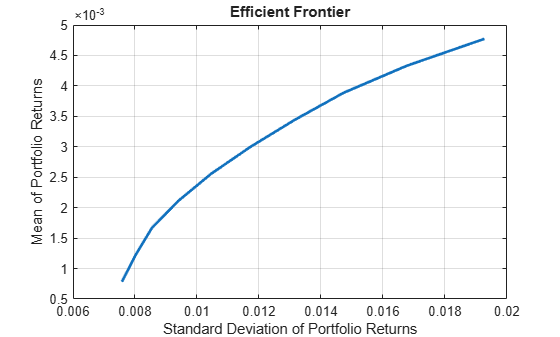# Mixed-Integer Mean-Variance Portfolio Optimization Problem

This example shows how to solve a mean-variance portfolio optimization problem with constraints in the number of selected assets or conditional (semicontinuous) bounds. To solve this problem, you can use a `Portfolio` object along with different mixed integer nonlinear programming (MINLP) solvers.

### Mean-Variance Portfolio

Load the returns data in `CAPMuniverse.mat`. Then, create a mean-variance `Portfolio` object with default constraints and a long-only portfolio whose weights sum to `1`. For this example, you can define the feasible region of weights $\mathit{X}$ as

`$\mathit{X}=\left\{\mathit{x}|\sum _{\mathit{i}=1}^{\mathit{n}}{\mathit{x}}_{\mathit{i}}=1,{\mathit{x}}_{\mathit{i}\text{\hspace{0.17em}}}\ge 0\right\}.$`

```% Load data load CAPMuniverse.mat % Create a mean-variance Portfolio object with default constraints p = Portfolio(AssetList=Assets(1:12)); p = estimateAssetMoments(p,Data(:,1:12)); p = setDefaultConstraints(p);```

Include binary variables for this scenario by setting conditional (semicontinuous) bounds. Conditional bounds are those such that ${\mathit{x}}_{\mathit{i}}=0$ or ${\mathit{x}}_{\mathit{i}}\ge {\ell }_{\mathit{i}}$. In this example, ${\ell }_{\mathit{i}}=0.1$ for all assets.

```% Set conditional bounds condLB = 0.1; condUB = 0.5; p = setBounds(p,condLB,condUB,BoundType="conditional");```

Use `estimateFrontier` to estimate a set of portfolios on the efficient frontier. The efficient frontier is a curve that shows the trade-off between the return and risk achieved by Pareto-optimal portfolios. For a given return level, the portfolio on the efficient frontier is the one that minimizes the risk while maintaining the desired return. Conversely, for a given risk level, the portfolio on the efficient frontier is the one that maximizes return while maintaining the desired risk level.

```% Compute efficient frontier pwgt = estimateFrontier(p)```
```pwgt = 12×10 0 0 0.1000 0.1253 0.1745 0.2236 0.2715 0.3327 0.4111 0.5000 0 0 0 0 0 0 0 0 0 0 0 0 0 0 0 0 0 0 0 0 0.1350 0 0 0 0 0 0 0 0 0 0 0 0 0 0 0 0 0 0 0 0.1000 0.1450 0.1406 0.1910 0.2344 0.2778 0.3200 0.3726 0.4415 0.5000 0.1000 0.1609 0.1642 0.2121 0.2415 0.2709 0.3085 0.2947 0.1474 0 0.2354 0.1875 0.1290 0 0 0 0 0 0 0 0 0 0 0 0 0 0 0 0 0 0.4296 0.4066 0.3662 0.3717 0.2496 0.1277 0 0 0 0 ⋮ ```
```% Compute risk and returns of the portfolios on the efficient frontier [rsk,ret] = estimatePortMoments(p,pwgt)```
```rsk = 10×1 0.0076 0.0080 0.0085 0.0094 0.0105 0.0117 0.0132 0.0147 0.0168 0.0193 ```
```ret = 10×1 0.0008 0.0012 0.0017 0.0021 0.0026 0.0030 0.0034 0.0039 0.0043 0.0048 ```

Plot the weights from the frontier estimation using `plotFrontier`. The resulting curve is piece-wise concave with vertical jumps (discontinuities) between the concave intervals.

```% Plot efficient frontier plotFrontier(p,pwgt)```### Changing MINLP Solvers

In the previous section, you use the default solver for `estimateFrontier`. However, you can solve mixed-integer portfolio problems using any of the three algorithms supported by `setSolverMINLP`: `OuterApproximation`, `ExtendedCP`, and `TrustRegionCP`. Furthermore, the `OuterApproximation` algorithm accepts an additional name-value argument (`ExtendedFormulation`) for `Portfolo` problems, which reformulates problems with quadratic functions to work in an extended space that usually decreases the computation time. All algorithms, including the extended formulation variation of the `OuterApproximation` algorithm, return the same values within numerical accuracy. The available solvers are:

• `OuterApproximation` — The default algorithm, which is robust and usually faster than `ExtenedCP`

• `OuterApproximation` with `ExtendedFormulation` set to `true` — A robust algorithm that is usually faster than other algorithms, but only supported for `Portfolio` object problems

• `ExtendedCP` — The most robust solver, but usually the slowest

• `TrustRegionCP` — The fastest algorithm, but one that is less robust and may provide suboptimal solutions

For more information on solvers for mixed-integer portfolio problems, see Choose MINLP Solvers for Portfolio Problems.

To change the MINLP solvers, use `setSolverMINLP`.

```% Select the extended formulation version of 'OuterApproximation' p_EOA = setSolverMINLP(p,'OuterApproximation',... ExtendedFormulation=true); pwgt_EOA = estimateFrontier(p_EOA); [rskEOA,retEOA] = estimatePortMoments(p_EOA,pwgt_EOA); % Select 'TrustRegionCP' as solver p_TR = setSolverMINLP(p,'TrustRegionCP'); pwgt_TR = estimateFrontier(p_TR); [rskTR,retTR] = estimatePortMoments(p_TR,pwgt_TR); % Select 'ExtendedCP' as solver using 'midway' cuts as 'CutGeneration' p_ECP = setSolverMINLP(p,'ExtendedCP','CutGeneration','midway'); pwgt_ECP = estimateFrontier(p_ECP); [rskECP,retECP] = estimatePortMoments(p_ECP,pwgt_ECP);```

Compare the returns and risks obtained by the portfolios on the efficient frontier from the different solvers. These are same within a numerical accuracy, where the absolute difference is $\le {10}^{-4}$.

```retTable = table(ret,retEOA,retTR,retECP,... 'VariableNames',{'OA','EOA','TR','ECP'})```
```retTable=10×4 table OA EOA TR ECP __________ __________ __________ __________ 0.00078336 0.00078336 0.00078334 0.00078348 0.0012267 0.0012267 0.0012267 0.0012268 0.00167 0.00167 0.00167 0.0016701 0.0021133 0.0021133 0.0021133 0.0021134 0.0025566 0.0025566 0.0025566 0.0025567 0.0029999 0.0029999 0.0029999 0.003 0.0034432 0.0034432 0.0034432 0.0034433 0.0038865 0.0038865 0.0038865 0.0038866 0.0043298 0.0043298 0.0043298 0.0043299 0.0047731 0.0047731 0.0047731 0.0047731 ```
```rskTable = table(rsk,rskEOA,rskTR,rskECP,... 'VariableNames',{'OA','EOA','TR','ECP'})```
```rskTable=10×4 table OA EOA TR ECP _________ _________ _________ _________ 0.0075778 0.0075778 0.0075778 0.0075778 0.0080234 0.0080234 0.0080235 0.0080236 0.0085488 0.0085488 0.0085488 0.008549 0.0094024 0.0094024 0.009405 0.0094025 0.010456 0.010456 0.010456 0.010456 0.011727 0.011727 0.011776 0.011728 0.013155 0.013155 0.013155 0.013155 0.014729 0.014729 0.014729 0.014729 0.016764 0.016764 0.016764 0.016764 0.019273 0.019273 0.019273 0.019273 ```
```% Compare risks from the different OuterApproximation formulations norm(rskTable.OA-rskTable.EOA,Inf) <= 1e-4```
```ans = logical 1 ```